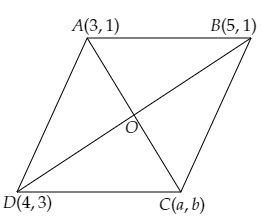# Points $A(3,1), B(5,1), C(a,b)$ and $D(4,3)$ are vertices of a parallelogram ABCD. Find the values of $a$ and $b$.√ҐвВђвАє

Given:

The points $A(3,1), B(5,1), C(a,b)$ and $D(4,3)$ are the vertices of a parallelogram $ABCD$.

To do:

We have to find the values of $a$ and $b$.

Solution:

Let the diagonals $AC$ and $BD$ bisect each other at $O$.Using the mid-point formula, we get,

$\mathrm{O}$ is the mid-point of $\mathrm{AC}$

The coordinates of $\mathrm{O}$ are $\left(\frac{3+a}{2}, \frac{1+b}{2}\right)$

Similarly,

$\mathrm{O}$ is the mid-point of $\mathrm{BD}$.

The coordinates of $\mathrm{O}$ are $\left(\frac{5+4}{2}, \frac{1+3}{2}\right)$

$=\left(\frac{9}{2}, \frac{4}{2}\right)$

$=\left(\frac{9}{2}, 2\right)$

On comparing, we get,

$\frac{9}{2}=\frac{3+a}{2}$

$\Rightarrow 3+a=9$

$\Rightarrow a=9-3=6$

$\frac{1+b}{2}=2$

$\Rightarrow b+1=4$

$\Rightarrow b=4-1=3$

The values of $a$ and $b$ are $6$ and $3$ respectively.

Updated on: 10-Oct-2022

100 Views